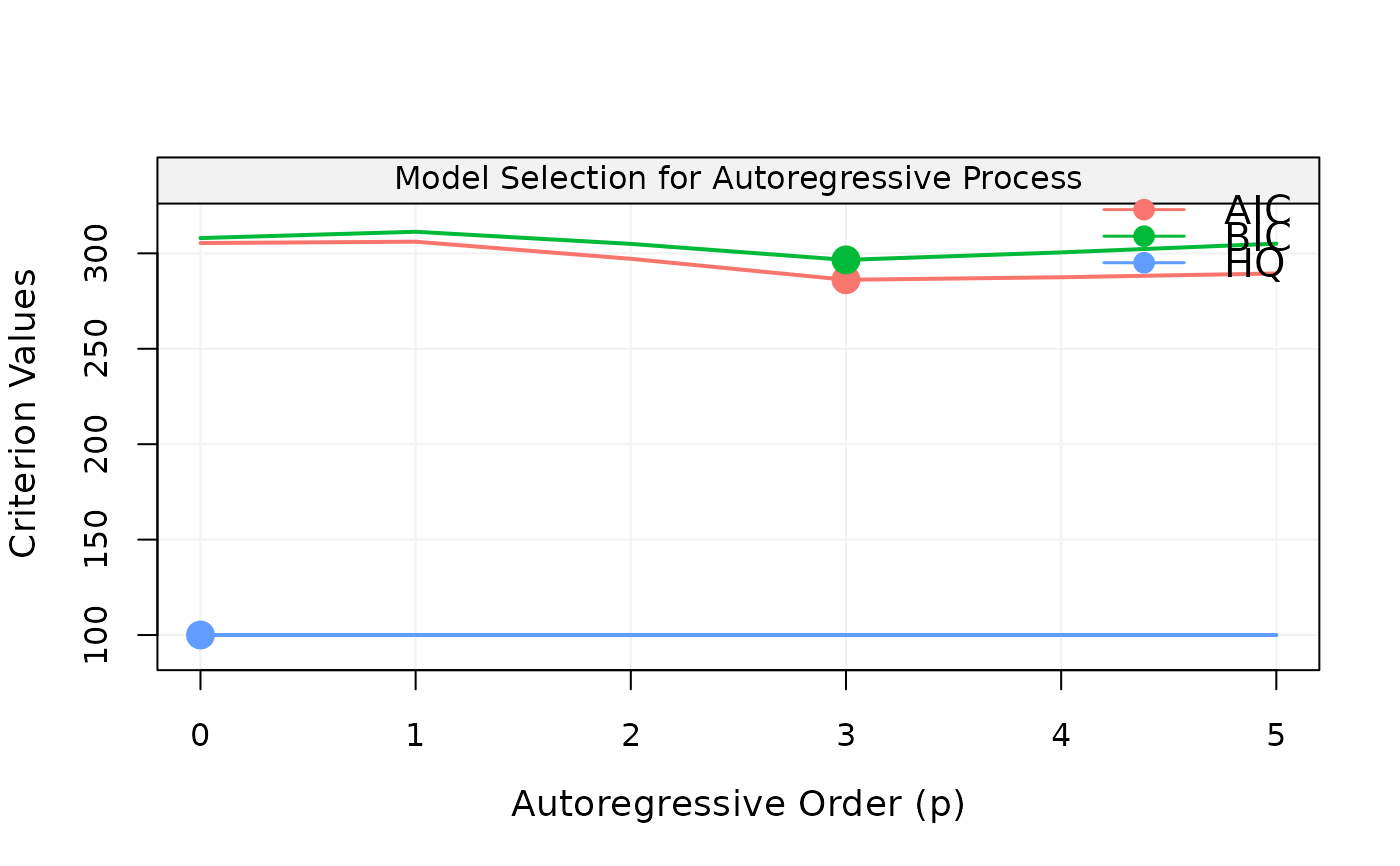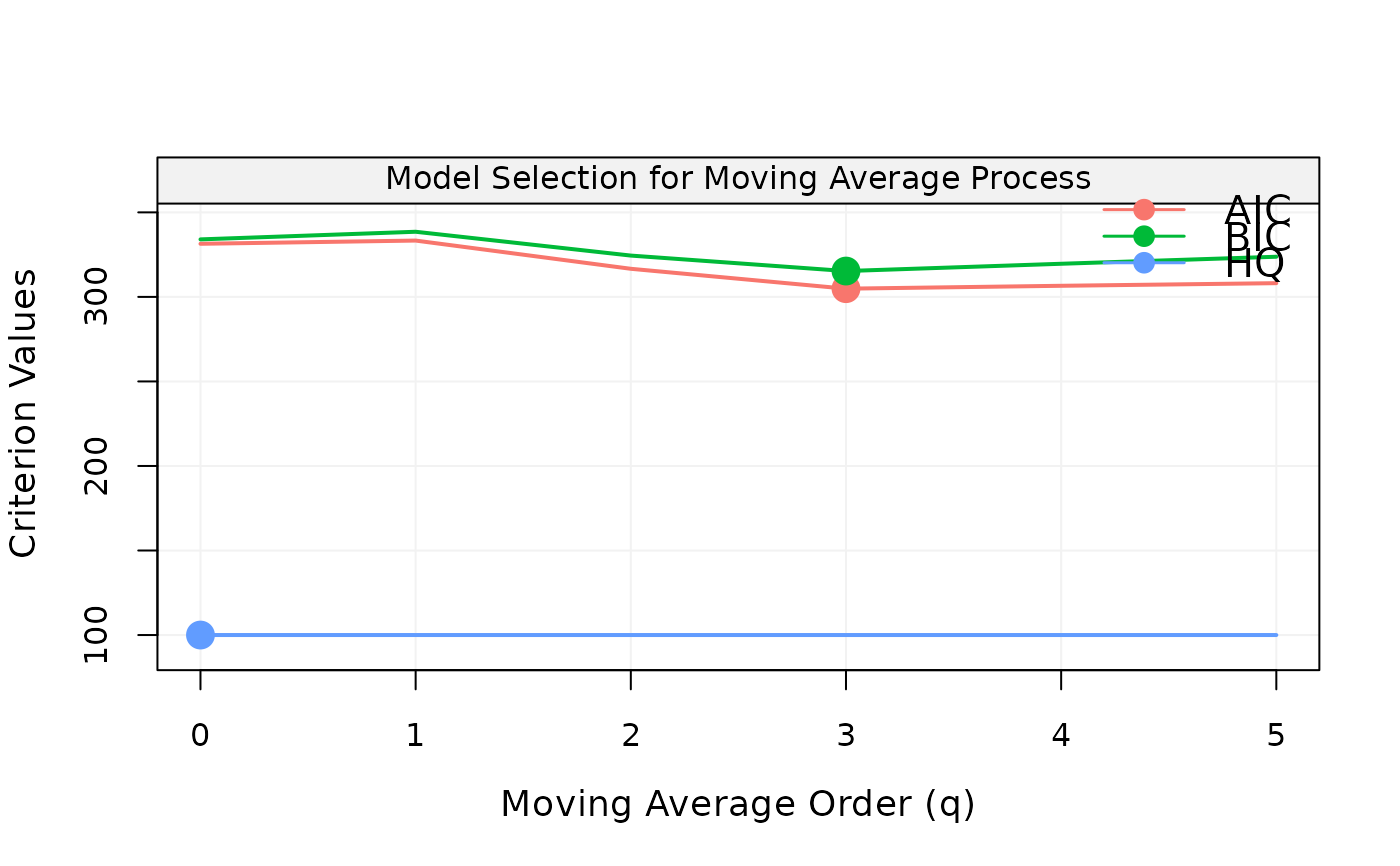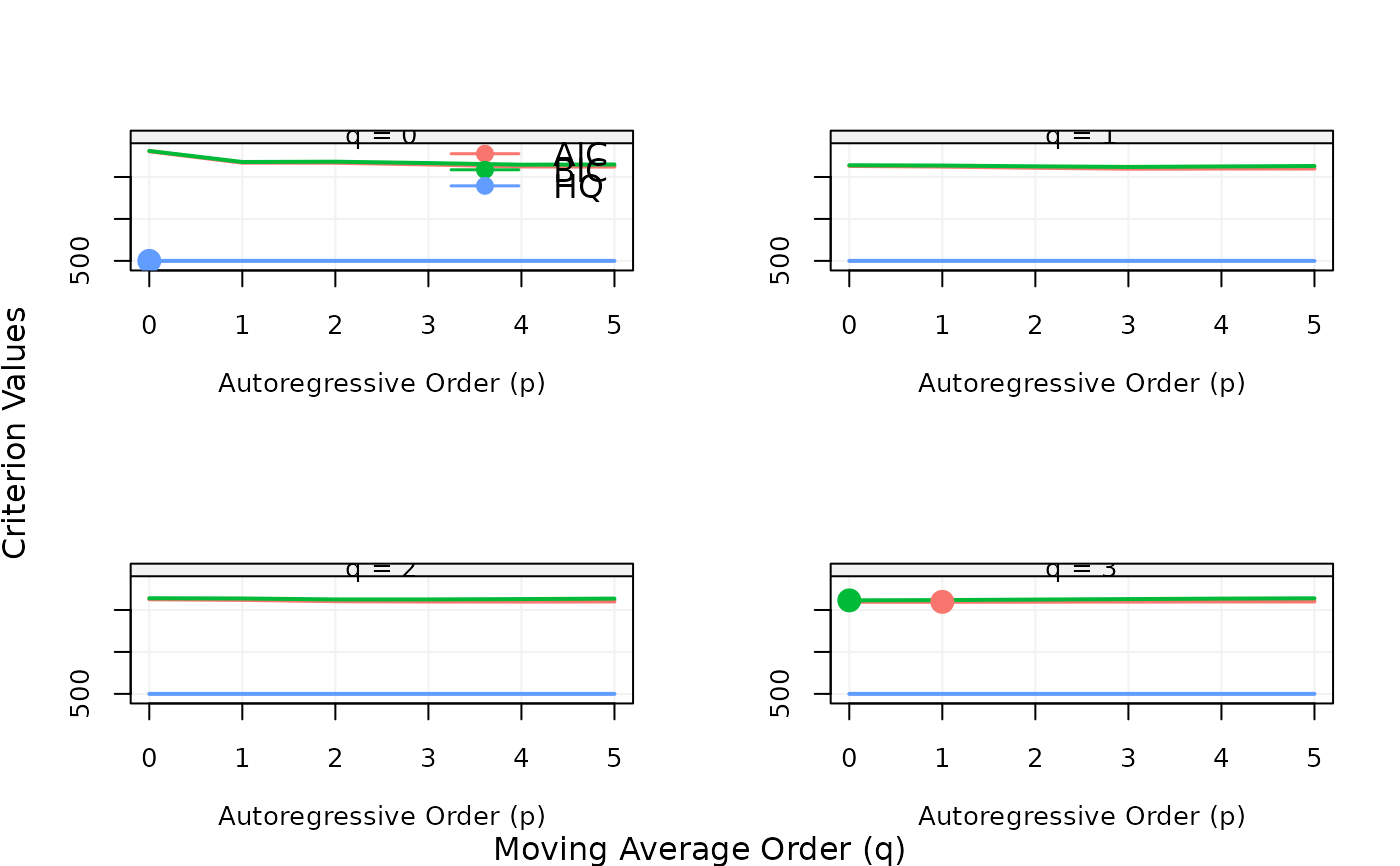This function performs model fitting and calculates the model selection criteria to be plotted.

select(model, Xt, include.mean = TRUE, criterion = "aic", plot = TRUE)

## Arguments

model

A time series model (only ARIMA are currently supported).

Xt

A vector of time series data.

include.mean

A boolean indicating whether to fit ARIMA with the mean or not.

criterion

A string indicating which model selection criterion should be used (possible values: "aic" (default), "bic", "hq").

plot

A boolean indicating whether a model selection plot is returned or not.

## Author

Stéphane Guerrier and Yuming Zhang

## Examples

set.seed(763)
Xt = gen_gts(100, AR(phi = c(0.2, -0.5, 0.4), sigma2 = 1))
select(AR(5), Xt, include.mean = FALSE)Xt = gen_gts(100, MA(theta = c(0.2, -0.5, 0.4), sigma2 = 1))
select(MA(5), Xt, include.mean = FALSE)Xt = gen_gts(500, ARMA(ar = 0.5, ma = c(0.5, -0.5, 0.4), sigma2 = 1))
select(ARMA(5,3), Xt, criterion = "hq", include.mean = FALSE)